## Trigonometry review for Statistics.com

Okay, trigonometry on the quick. Let me know if I leave out too many things.

First, trigonometry is super useful as it helps answer questions related to both triangles and circles. Since engineers dating back to the Greeks used these in design, clearly the concepts are fundamental. Let's recall on key fact, known to Pythagorus:

For a right triangle with sides a,b, and c (where c is the hypotenuse) the sides are related by the formula


$c^2 = a^2 + b^2$

Hopefully that is familiar. When we are done, we will be able to modify this for non right triangles.

Okay, let's start with triangles, in fact right triangles. Here is a picture:

plot(c(0, 4, 0, 0), c(0, 0, 3, 0), type = "l")
text(c(0.25, 2, 2, 3.5), c(1.5, 0.25, 2, 0.25), labels = c("b", "a",
"c", "theta"))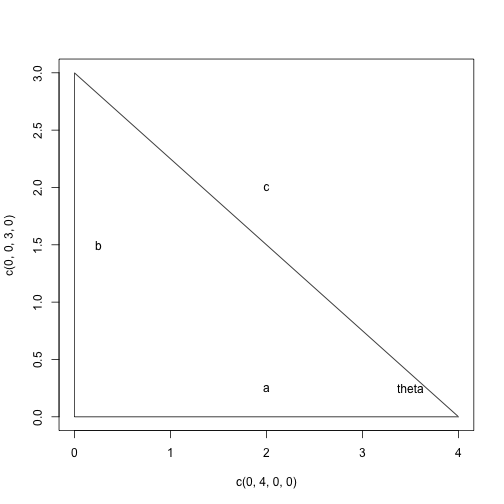The triangle is a 3-4-5 right triangle, and notice 9 + 16 = 25, as Pythagorus would like me to point out. Now clearly, the 4 variables a,b,c and theta are related, trignometry answers how. Pythagorus relates a,b, and c but not theta. The fundamental definitions of trignometry just assign a function to describe the relationship.

So, for now, let's assume theta is between 0 and pi/2 (90 degrees, but we are working with radians). Then with the figure as drawn we define two new functions $$\sin(\theta)$$ and $$\cos(\theta)$$ by:

$\sin(\theta) = b/c = \text{opposite}/\text{hypotenuse} \quad\text{and}\quad \cos(\theta) = a/c = \text{adjacent}/\text{hypotenuse}$

So with these two new functions we can tie together all four variables (we introduced “opposite”, “adjacent” and “hypotenuse” as the labels otherwise can be arbitrary). Now really only one of these functions is necessary, as you can see that with some substitution and Pythagorus, you can write the cosine squared in terms of sine squared as:

$\cos(x)^2 + \sin(x)^2 = 1$
but let's hold off on that, as it becomes more clear with circles. Now, there are 4 more functions that people define, 1 that is useful, 3 more that are just reciprocals and I find distracting (a personal taste). The useful one is the tangent:

$\tan(\theta) = \sin(\theta)/\cos(\theta) = b/a = \text{opposite}/\text{adjacent}$

Okay, armed with that generally you next get taught that there are certain angles where geometry can help you find out the answers for given angles. Lets do $$\pi/4$$ or 45 degrees:

d <- 0.05
plot(c(0, 1, 0, 0), c(0, 0, 1, 0), type = "l")
text(c(d, 0.5, 0.5, 1 - 3 * d), c(0.5, d, 0.65, d), labels = c("1",
"1", "sqrt(2)", "pi/4"))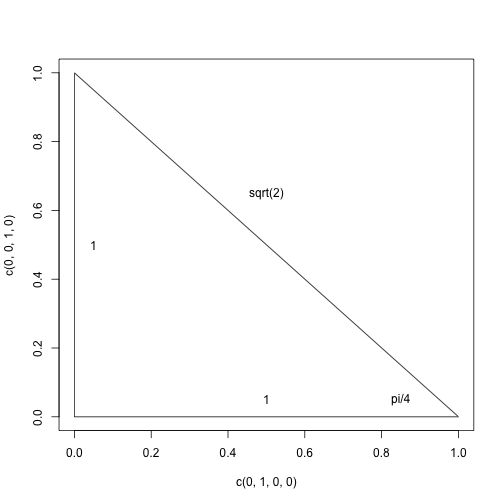From this you get

$\sin(\pi/4) = 1/\sqrt(2), \quad \cos(\pi/4) = 1/\sqrt(2), \quad \tan(\pi/4) = 1$

Another easy one is $$\theta=0$$. For this adjacent and hypotenuse are the same and opposite is 0, so we have

$\sin(0) = 0, \quad \cos(0) = 1, \quad \tan(0) = 0$

By contrast, if $$\theta = \pi/2$$ we have adjacent is 0 and the opposite and hypotenuse are equal giving:

$\sin(0) = 1, \quad \cos(0) = 0, \quad \tan(0) = \text{undefined}$

One can also get values for 30 and 60 degrees ($$\pi/6, \pi/3$$).

As for other values, you can a) use a calculator or b) use some tricks. When you saw this earlier, likely you spent some time with some of the double angle formulas. Here is one:

$\sin(2 \theta) = 2 \sin(\theta) \cos(\theta)$

With this, you can rearrange to find the sine of 15 degrees in terms of the sin of 30 degrees … The double angle formulas are related to the sum formulas which allow one to express the sine of the sum of two angles in terms of the sine and cosine of the angles separately.

Okay, how does this help? Well, a simple question might be to figure out how tall something is. Say you estimate you are 100 feet from an object and that object stands 11 degrees tall. How high is that object?

Well, here is a picture:

plot(c(0, 100, 0, 0), c(0, 0, 100 * tan(11 * pi/180), 0), type = "l")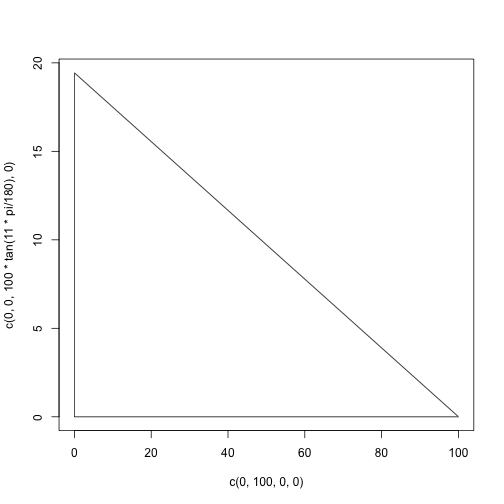The desired side is the opposite, the adjacent is 100 and the angle is 11 degrees of $$11 \cdot \pi/180$$ radian. The answer then is given by

$\tan(\theta) = x/100$

Or

100 * tan(11 * pi/180)

##  19.44


## Law of Sines, Law of Cosines

Two useful formulas relating sines and cosines are the law of sines and law of cosines. For this, we don't need a right triangle, but we do need to give names (a, b, and c). The angle opposite a side is given the same name, only upper case (A, B, C). Then the law of sines is

$\sin(A)/a = \sin(B)/b = \sin(C)/c$

Check that this is true for a right triangle. What makes it really useful is that it is true for any triangle.

The law of cosines extends the Pythagorus theorem:

$c^2 = a^2 + b^2 - 2ab \cos(C)$

Note for right triangles, $$\pi/2$$, so $$\cos(C) = 0$$ and we get the pythagorus theorem right back at us. Again, this is always true though.

Here is an application: A carpenter cut a triangle with sides 4, 7 and hypotenuse 8. Did he cut a right triangle? If not, what is the angle opposite the length of side 8?

Well, since

a = 4
b = 7
c = 8
c^2 - (a^2 + b^2)

##  -1


is not zero, it is not a right triangle. In fact, we can solve for the cosine of C to get it is equal to:

c^2 - (a^2 + b^2))/(-2*a*b)


That isn't quite so helpful. A more natural question is what angle does this correspond to? Well, that would require us to know the inverse function. Well, let's say since cosine is monotonic from 0 to $$\pi$$ an inverse function can be defined for that domain, and it is programmed into R:

acos(0.01785714)

##  1.553


In radians, it is hard to tell that value. Let's see in degrees:

acos(0.01785714) * 180/pi

##  88.98


May be time to find a new carepenter?

## Circles

Now triangles work well to introduce the topic, but they work best when theta is between 0 and 90 degrees. The sine and cosine functions are much more general – they are defined for all thetat. A better way to define them is in terms of the unit circle, the circle of radius 1 centered at the origin (aka $$x^2 + y^2=1$$):

x <- seq(-1, 1, length = 100)
plot(x, sqrt(1 - x^2), type = "l", ylim = c(-1, 1))
lines(x, -sqrt(1 - x^2))
theta <- pi/3
x <- cos(theta)
y <- sin(theta)
points(x, y, cex = 2, pch = 16)
lines(c(0, x), c(0, y), lty = 3)
text(0.15, 0.1, labels = "theta")
text(x + 0.15, y, labels = "(x,y)")
abline(v = 0, col = "gray")
abline(h = 0, col = "gray")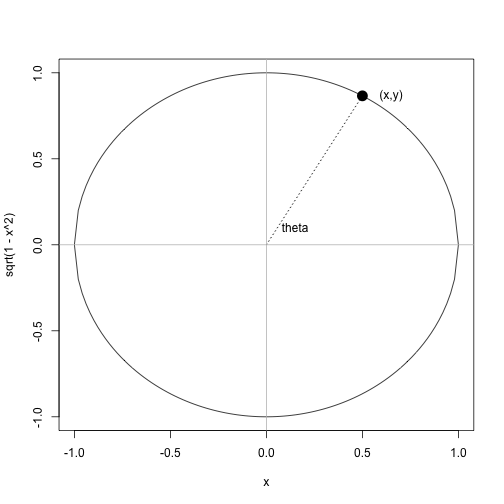In the figure, you can imagine a triangle (in reverse image to our previous) with the hypotenuse 1, adjacent x and opposite y. So we get from our previous definitions, for the unit circle:

$\sin(\theta) = y, \quad \cos(\theta) = x, \quad \tan(\theta) = y/x$

Why is that useful, well this applies for any theta value. If we measure theta from the x axis, positive being counter clockwise, then this can define the sine and cosine for any angle. Clearly they are periodic – they repeat – as you will get the same thing again after the angle has gone once around the circle. This manifests itself in formulas like:

$\sin(\theta + 2\pi) = \sin(\theta)$

There are many others, including some that relate the sin and cosine after shifting the angle.

If instead of a unit circle, you had a circle with radius r things change a bit, as the hypotenuse is r, not 1, so will appear in the formulas. Usually these are related in this manner:

$x = r \cos(\theta), \quad y = r \sin(\theta).$

For the unit circle there is one unlabeled value: the arc length from the point (1,0) to the point (x,y). In the figure this is more than 0 and less than a quarter trip around the circle (which is $$2\pi/4 = \pi/2$$, as the circumference of the unit circle is $$2\pi$$). In fact, if you know that angle in degrees you can figure it out by rations:

$\text{angle in degrees}/360 = x/(2\pi)$
Solving gives, $$x = \text{angle} \cdot \pi/180$$, or the conversion to radians. So radians are secretly a distance – something physical.

## The graphs

The graph of the $$\sin(\theta)$$ has some properties you can glean from the unit circle:

• it is 0 at 0 (as y is 0 for 0 radians).
• it is 1 at $$\pi/2$$ (the top of the circle)
• it is 0 at $$\pi$$ (or 180 degrees, as here y is 0
• it is -1 at $$3\pi/2$$ (the bottom of the circle)
• it is negative when $$\pi < \theta < 2\pi$$ (the bottom of the circle)
• it repeats itself after $$2\pi$$.

So let's see it graphed from 0 to $$4\pi$$, or two periods:

theta <- seq(0, 4 * pi, length.out = 1000)
plot(theta, sin(theta), type = "l")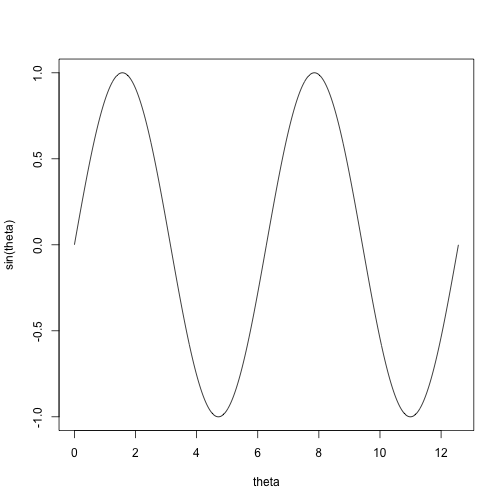Try to verify all of the above.

The graph of the cosine is similar, though is some sense it is ahead by 90 degrees (it starts at 1). Here I plot both:

plot(theta, cos(theta), type = "l", lwd = 2)
lines(theta, sin(theta), col = "gray90")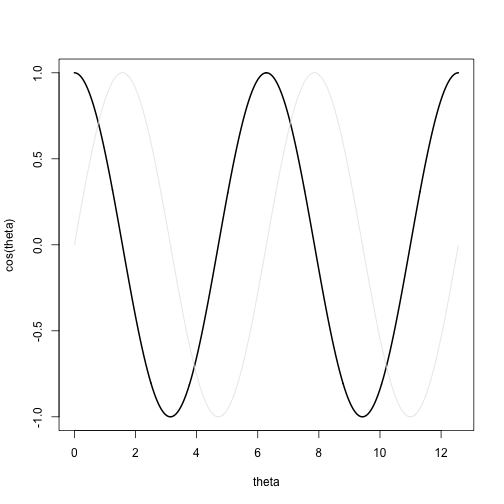The tangent function has asymptotes, as every so often the divisor is 0. Here is one period

theta <- seq(-pi/2 + 0.1, pi/2 - 0.1, length = 1000)
plot(theta, tan(theta), type = "l")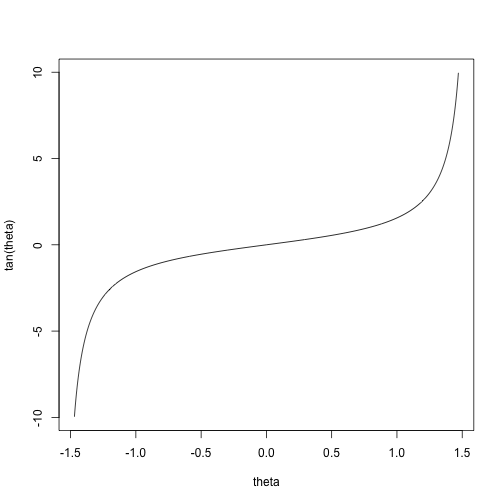Related to graphs are the concepts of shifting and stretching. Recall, if you know the graph of $$f(x)$$, then you know what the graph of $$g(x) = a + b f(c(x + d))$$. (The value of $c$ changes the x axis, d moves on $x$ axis, b changes amplitud and a moves up or down.

Here is a familiar thing related to this.

The amount of daylight follows a sine curve (well, the earth more or less moves in a cicular manner and the amont of light depends on that). Let's say the curve has a peak in summer of 16, a minimum in winter of 8 and a period of 365 days

So we need to
a) change the period to 365 days
b) shift up by 12
c) change amplitude by 4
d) shift over so the graph starts on March 21st (day 31 + 38 + 21)

First the period: we need to stretch the x axis from 2pi to 365, let's do $$c = 2\pi/365$$. Then when $$x$$ is 365, the value $$cx$$ is $\latex 2\pi$ or one period.

Next the shift, we need to move to the right by 80=31 + 28 + 21. Let's try graphing:

x <- seq(0, 365, length = 1000)
plot(x, sin((2 * pi/365) * (x - 80)), type = "l")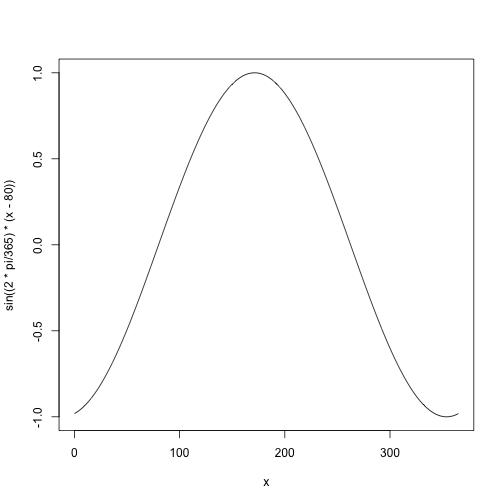Now to shift up by 12 and change the amplitude to 4 (as it goes from 8 to 16):

x <- seq(0, 365, length = 1000)
plot(x, 12 + 4 * sin((2 * pi/365) * (x - 80)), type = "l")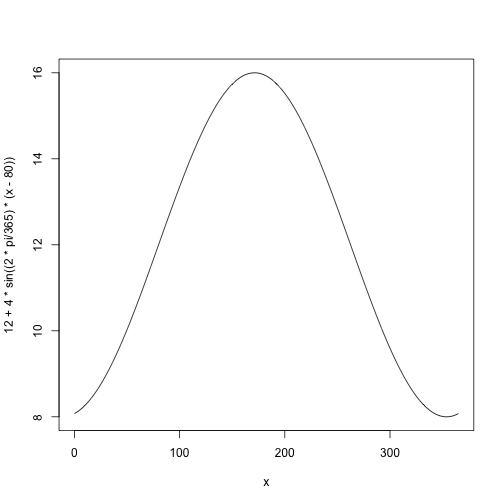Less familiar, but no less useful is the decomposition of things into Fourier sums – sums of transformed sines and cosines. Most nice functions admit a representation that can be used to digitize a function.